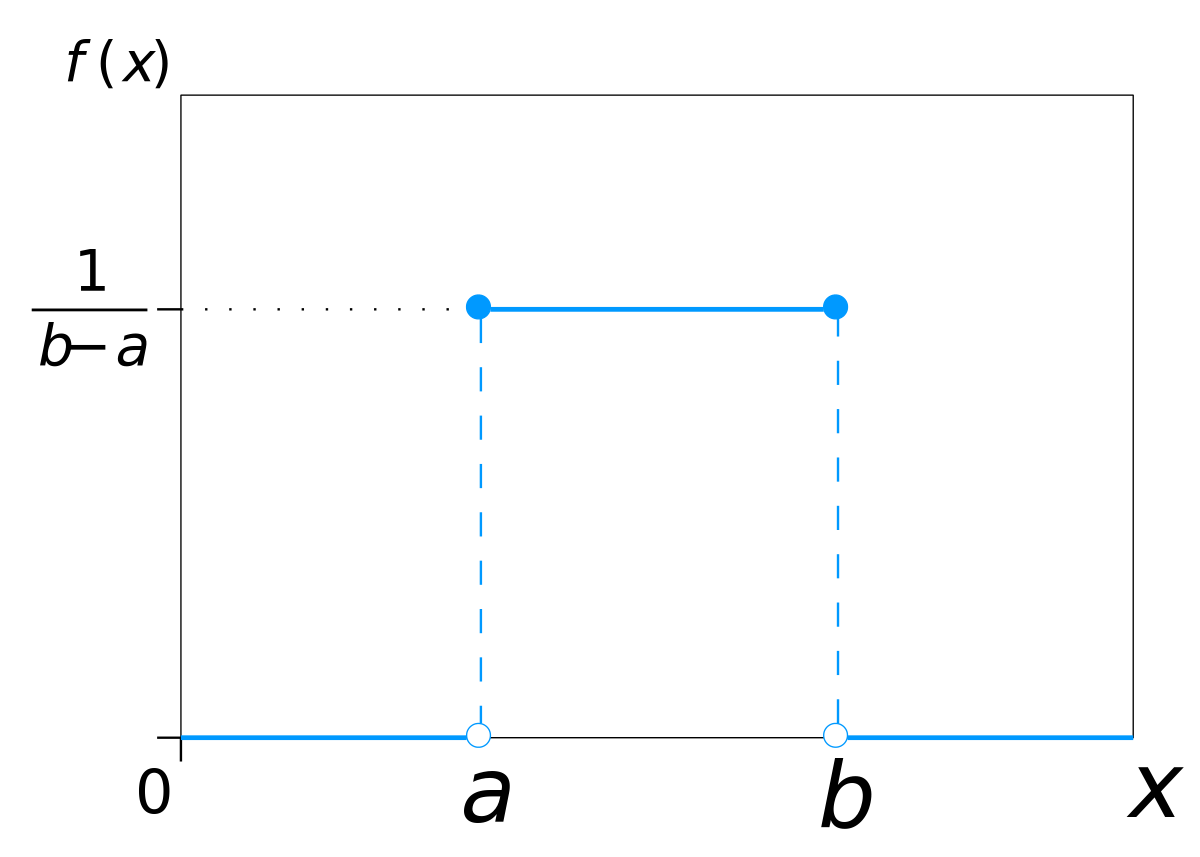# Weight Initialization Methods - Class review

Last updated on：a year ago

A well chose initialization of filter weights can speed up your learning systems. I want to make a summary of some advanced weight initialization methods in this blog.

# What is Initialization?

When you train your learning systems, you should first set the parameters of the systems. Better values of parameters can later be obtained by updating.

A well-chosen initialization can:

• Speed up the convergence of gradient descent

• Increase the odds of gradient descent converging to a lower training (and generalization) error

Initializing weights to very large random values does not work well.

# Zeros initialization

Zeros initialization means you initialize your learning systems rate with 0 value, which is harmful, which leads to network failing break symmetry and $n^{[l]} = 1$.

Symmetry breaking

A phenomenon in which (infinitesimally) small fluctuations acting on a system crossing a critical point decide the systems’ fate, by determining which branch of a bifurcation is taken.

# Random initialization

## Gaussian distributions initialization

$$Var[W^i] = \sigma^2$$

$$W\sim \mathcal{N} (0, \sigma^2)$$

N means normal distribution.

• Symmetry is broken so long as $W^{[l]}$ is initialized randomly.

Shortcoming

• As the result of fixed standard deviations, very deep models (> 8 conv layers) have difficulties to converge.

## Xavier initialization

$$Var[W^i] = \frac{2}{n_i + n_{i+1}}$$

$$W\sim U[-\frac{\sqrt{6}}{\sqrt{n_j + n_{j+1}}}, \frac{\sqrt{6}}{\sqrt{n_j + n_{j+1}}}]$$

U means continuous uniform distribution.

$$f(n) = \begin{cases} \frac{1}{b-a}, & \text{for a\leq x\leq b} \\ 0, & \text{for x<a or x>b} \end{cases}$$## He initialization

$$Var[W^i] =\sqrt{\frac{2}{n_l}}$$

$$W\sim \mathcal{N} (0, \sqrt{\frac{2}{n_l}})$$

Multiply $\sqrt{\frac{2}{layers-dims[l - 1]}}$ when initializing $W^{[l]}$

Xavier initialization vs. He initialization

The former is for linear activation functions only, but the latter is also valid by taking ReLU/PReLU into account.

# Reference

 He, K., Zhang, X., Ren, S. and Sun, J., 2015. Delving deep into rectifiers: Surpassing human-level performance on imagenet classification. In Proceedings of the IEEE international conference on computer vision (pp. 1026-1034).

 Glorot, X. and Bengio, Y., 2010, March. Understanding the difficulty of training deep feedforward neural networks. In Proceedings of the thirteenth international conference on artificial intelligence and statistics (pp. 249-256). JMLR Workshop and Conference Proceedings.